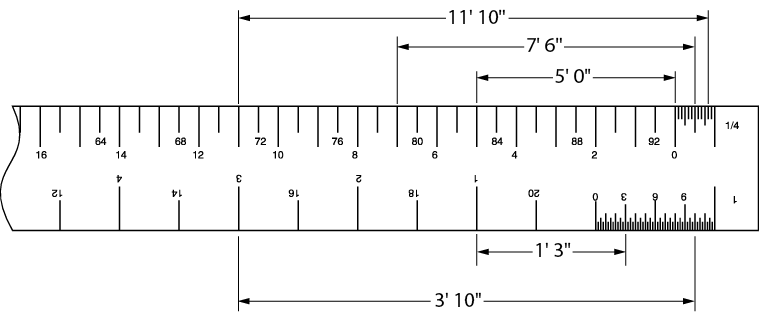# Untitled Page 19

Architect’s (imperial) scales

Traditional architectural measurements of length are written very precisely in feet and inches using the appropriate symbols for feet and inches separated by a dash (e.g., 4'-3 ½" and 7'-0"). This is the way that all imperial measurements are written on construction drawings.

Listed below are the scales found on the architect’s triangular scale ruler.

1. 3/32" =1'-0"
2. 3/16" = 1'-0"
3. 1/8" = 1'-0"
4. ¼" = 1'-0"
5. ¾" = 1'-0"
6. 3/8" = 1'-0"
7. 1" =1'-0"
8. ½" = 1'-0"
9. 1½" = 1'-0"
10. 3" = 1'-0"
11. 1" = 1" (full size—use the scale labelled 16)

Figure 3 shows one face of an architect’s imperial triangular scale ruler. There are two edges on each face and each edge contains two scales that run in opposite directions. At each end of an edge, a number or fraction indicates the distance in inches that represents one foot. The top edge is in eighths of an inch from left to right, and in quarters of an inch from right to left. Note that the 1/8" scale from 0 to the right end represents 95 feet, and the ¼" scale from 0 to the left end represents 47 feet.1. One face of an architect’s ruler (NTS)

At each end, between the zero and the number indicating scale, the length representing one foot is subdivided into 6, 12, 24, or more parts to indicate inches and, in some scales, fractions of an inch. For example, each of the six marks on the 1/8" scale represents two inches, while each mark equals a quarter of an inch on the 1" reduction scale and one inch on the ¼" scale.

Now look at the 1½" scale in Figure 4. The subdivided unit is divided into inches and fractions of an inch. Reading left from the zero, notice the figures 3, 6, and 9, which represent measurements of 3", 6", and 9". From the zero to the first long mark represents 1". Between the zero and the one-inch mark there are four spaces, each of which represent one-quarter of an inch.2. Units in an architect’s scale ruler (NTS)

Piping drawings usually use a 1/8" scale for larger buildings, a ¼" scale for smaller buildings and houses, and a ½" scale for details. Each drawing will state in the title box the scale that is used. Sometimes when special details are given, the scale is placed directly under the detail.

To draw or measure a length to scale, first find the edge of the ruler containing the scale. One end of the length will rest exactly on one of the foot marks of the scale, and the other end should rest either on the zero marker or somewhere on the inch subdivision of the scale. The length can then be marked and drawn or read off from a drawing.

Figures 5 and 6 demonstrate this manner of reading dimensions from four of the ratios on the architect’s scale.3. Reading dimensions using an architect’s ruler (NTS)4. Reading dimensions using an architect’s ruler (NTS)Architectural units have feet divided into inches, whereas engineering units divide feet into tenths and hundredths. Engineers’ scales are not used to make piping drawings.